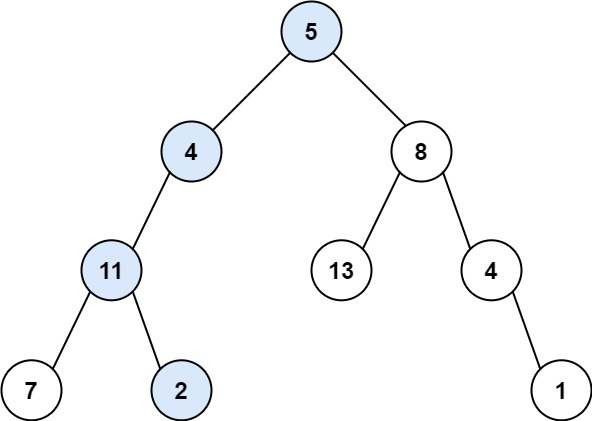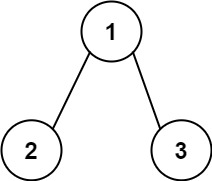0112. 路径总和

# 0112. 路径总和#

• 标签：树、深度优先搜索
• 难度：简单

## 题目大意 #

• 树中节点的数目在范围 $[0, 5000]$ 内。
• $-1000 \le Node.val \le 1000$。
• $-1000 \le targetSum \le 1000$。

• 示例 1：1 2 3  输入：root = [5,4,8,11,null,13,4,7,2,null,null,null,1], targetSum = 22 输出：true 解释：等于目标和的根节点到叶节点路径如上图所示。 
• 示例 2：1 2 3 4 5 6  输入：root = [1,2,3], targetSum = 5 输出：false 解释：树中存在两条根节点到叶子节点的路径： (1 --> 2): 和为 3 (1 --> 3): 和为 4 不存在 sum = 5 的根节点到叶子节点的路径。 

## 解题思路 #

### 思路 1：递归遍历 #

1. 定义一个递归函数，递归函数传入当前根节点 root，目标节点和 targetSum，以及新增变量 currSum（表示为从根节点到当前节点的路径上所有节点值之和）。
2. 递归遍历左右子树，同时更新维护 currSum 值。
3. 如果当前节点为叶子节点时，判断 currSum 是否与 targetSum 相等。
1. 如果 currSumtargetSum 相等，则返回 True
2. 如果 currSum 不与 targetSum 相等，则返回 False
4. 如果当前节点不为叶子节点，则继续递归遍历左右子树。

### 思路 1：代码 #

  1 2 3 4 5 6 7 8 9 10 11 12  class Solution: def hasPathSum(self, root: TreeNode, targetSum: int) -> bool: return self.sum(root, targetSum, 0) def sum(self, root: TreeNode, targetSum: int, curSum:int) -> bool: if root == None: return False curSum += root.val if root.left == None and root.right == None: return curSum == targetSum else: return self.sum(root.left, targetSum, curSum) or self.sum(root.right, targetSum, curSum) 

### 思路 1：复杂度分析 #

• 时间复杂度：$O(n)$，其中 $n$ 是二叉树的节点数目。
• 空间复杂度：$O(n)$。递归函数需要用到栈空间，栈空间取决于递归深度，最坏情况下递归深度为 $n$，所以空间复杂度为 $O(n)$。